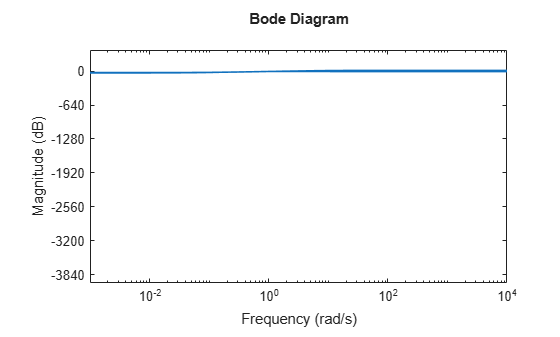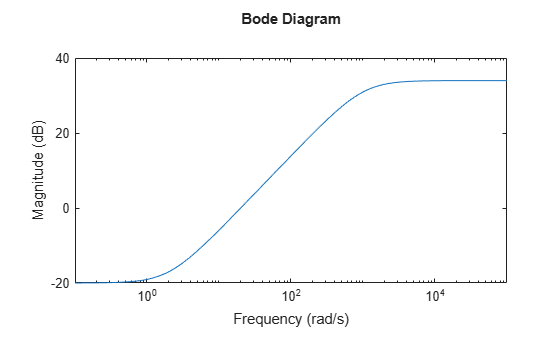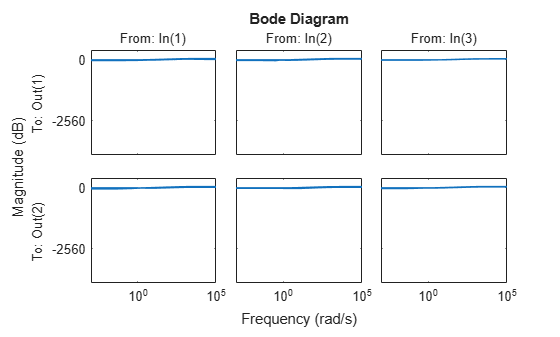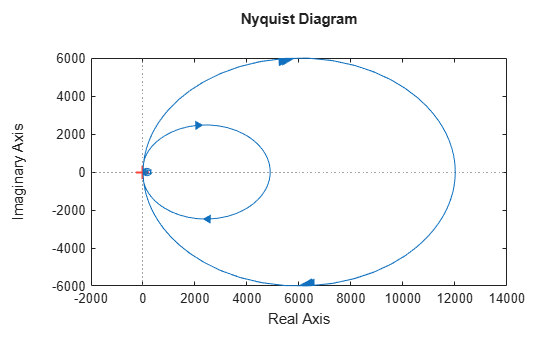# ultidyn

Uncertain linear time-invariant dynamics

## Description

`ultidyn` objects represent uncertain linear time-invariant dynamics whose only known attributes are bounds on their frequency response. Combine `ultidyn` objects with other dynamic system models and uncertain elements to model systems with uncertain dynamics, represented by `uss` or `genss` models.

## Creation

### Syntax

``H = ultidyn(name,iosize)``
``H = ultidyn(name,iosize,Name,Value)``

### Description

example

````H = ultidyn(name,iosize)` creates a `ultidyn` object modeling uncertain dynamics with a gain bound of 1 and output and input dimensions determined by `iosize`. ```

example

````H = ultidyn(name,iosize,Name,Value)` sets properties using one or more name-value arguments. For example, to set the gain bound to 0.1, set `"Bound"` to 0.1. ```

### Input Arguments

expand all

Output and input dimensions of the uncertain dynamics, specified as a scalar value or a vector of the form `[Ny Nu]`, where `Ny` is the number of outputs and `Nu` is the number of inputs. If you specify a scalar value `N`, then `H` has `N` outputs and inputs.

## Properties

expand all

Form in which to express bound on the frequency response of the uncertain dynamics, specified as either `'GainBounded'` or `'PositiveReal'`. This value determines the meaning of the `Bound` property as follows:

• `'GainBounded'` — The limit on the frequency response is expressed as an upper bound on the absolute gain, such that ```abs(H) <= H.Bound``` (for SISO dynamics) or ```hinfnorm(H) <= H.Bound``` (for MIMO dynamics) at all frequencies.

• `'PositiveReal'` — The limit on the frequency response is expressed as a lower bound on the real part, such that ```Real(H) >= H.Bound``` (for SISO dynamics) or `H + H' >= 2*H.Bound` (for MIMO dynamics) at all frequencies.

Bound on frequency response, specified as a scalar value. The meaning of this value depends on the value of the `Type` property, as described in description of that property. The default value also depends on how you set the `Type` property on object creation.

• If you do not specify `Type` or if you set ```Type = 'GainBounded'``` on object creation, then the default value is `Bound = 1`, meaning that the maximum absolute gain of the uncertain dynamics is 1 at all frequencies.

• If you set `Type = 'PositiveReal'` on object creation, then the default value is `Bound = 0`, meaning that the real part of the frequency response is greater than or equal to 0 at all frequencies.

Number of states in random samples of the block, specified as an integer. Some analysis commands such as `usample` and `bode` take random samples of uncertain dynamics. This property determines the number of states in the samples. For more information about how sampling of dynamic uncertainty works, see Generate Samples of Uncertain Systems.

Maximum frequency of random samples, specified as a positive scalar value. Randomly sampled uncertain dynamics are no faster than the specified value.

Nominal value, specified as a state-space model with the output and input dimensions specified by `iosize`. The nominal value of a `ultidyn` block is always 0 regardless of the uncertain dynamics the block represents.

Block simplification level, specified as `'basic'`, `'full'`, or `'off'`. In general, when you combine uncertain elements to create uncertain state-space models, the software automatically applies techniques to eliminate redundant copies of the uncertain elements. (See `simplify`.) Use this property to specify the simplification to apply when you use model arithmetic or interconnection techniques with the uncertain block.

• `'basic'` — Apply the elementary simplification method after each arithmetical or interconnection operation.

• `'full'` — Apply techniques similar to model reduction.

• `'off'` — Perform no simplification.

Name of uncertain element, specified as a string or character vector and stored as a character vector. When you create an uncertain state-space (`uss` or `genss`) model using uncertain control design blocks, the software tracks the blocks using the name you specify in this property, not the variable name in the MATLAB® workspace. For example, if you create a `ultidyn` block using `H = ultidyn("Delta",2)`, and combine the block with a numeric LTI model, the `Blocks` property of the resulting `uss` model lists the uncertain control design block `Delta`.

Names of input channels, specified as:

• A character vector or string — For single-input models

• A cell array of character vectors or a string array — For models with two or more inputs

• `''` — For inputs without specified names

You can use automatic vector expansion to assign input names for multi-input models. For example, if `sys` is a two-input model, you can specify `InputName` as follows.

`sys.InputName = 'controls';`

The input names automatically expand to `{'controls(1)';'controls(2)'}`.

You can use the shorthand notation `u` to refer to the `InputName` property. For example, `sys.u` is equivalent to `sys.InputName`.

Input channel names have several uses, including:

• Identifying channels on model display and plots

• Extracting subsystems of MIMO systems

• Specifying connection points when interconnecting models

If you specify `InputName` using a string or string array, such as `"voltage"`, the input name is stored as a character vector, `'voltage'`.

Units of input signals, specified as:

• A character vector or string — For single-input models

• A cell array of character vectors or string array — For models with two or more inputs

• `''` — For inputs without specified units

Use `InputUnit` to keep track of the units each input signal is expressed in. `InputUnit` has no effect on system behavior.

If you specify `InputUnit` using a string, such as `"voltage"`, the input units are stored as a character vector, `'voltage'`.

Example: `'voltage'`

Example: `{'voltage','rpm'}`

Input channel groups, specified as a structure where the fields are the group names and the values are the indices of the input channels belonging to the corresponding group. When you use `InputGroup` to assign the input channels of MIMO systems to groups, you can refer to each group by name when you need to access it. For example, suppose you have a five-input model `sys`, where the first three inputs are control inputs and the remaining two inputs represent noise. Assign the control and noise inputs of `sys` to separate groups.

```sys.InputGroup.controls = [1:3]; sys.InputGroup.noise = [4 5];```

Use the group name to extract the subsystem from the control inputs to all outputs.

`sys(:,'controls')`

Example: `struct('controls',[1:3],'noise',[4 5])`

Names of output channels, specified as:

• A character vector or string— For single-output models

• A cell array of character vectors or string array — For models with two or more outputs

• `''` — For outputs without specified names

You can use automatic vector expansion to assign output names for multi-output models. For example, if `sys` is a two-output model, you can specify `OutputName` as follows.

`sys.OutputName = 'measurements';`

The output names automatically expand to `{'measurements(1)';'measurements(2)'}`.

You can use the shorthand notation `y` to refer to the `OutputName` property. For example, `sys.y` is equivalent to `sys.OutputName`.

Output channel names have several uses, including:

• Identifying channels on model display and plots

• Extracting subsystems of MIMO systems

• Specifying connection points when interconnecting models

If you specify `OutputName` using a string, such as `"rpm"`, the output name is stored as a character vector, `'rpm'`.

Units of output signals, specified as:

• A character vector or string — For single-output models

• A cell array of character vectors or string array — For models with two or more outputs

• `''` — For outputs without specified units

Use `OutputUnit` to keep track of the units each output signal is expressed in. `OutputUnit` has no effect on system behavior.

If you specify `OutputUnit` using a string, such as `"voltage"`, the output units are stored as a character vector, `'voltage'`.

Example: `'voltage'`

Example: `{'voltage','rpm'}`

Output channel groups, specified as a structure where the fields are the group names and the values are the indices of the output channels belonging to the corresponding group. When you use `OutputGroup` to assign the output channels of MIMO systems to groups, you can refer to each group by name when you need to access it. For example, suppose you have a four-output model `sys`, where the second output is a temperature, and the rest are state measurements. Assign these outputs to separate groups.

```sys.OutputGroup.temperature = ; sys.OutputGroup.measurements = [1 3 4];```

Use the group name to extract the subsystem from all inputs to the measurement outputs.

`sys('measurements',:)`

Example: `struct('temperature',,'measurement',[1 3 4])`

Text notes about the model, specified as a string or character vector. The property stores whichever of these two data types you provide. For instance, suppose that `sys1` and `sys2` are dynamic system models. You can set their `Notes` properties to a string and a character vector, respectively.

```sys1.Notes = "sys1 has a string."; sys2.Notes = 'sys2 has a character vector.'; sys1.Notes sys2.Notes```
```ans = "sys1 has a string." ans = 'sys2 has a character vector.' ```

You can also specify `Notes` as string array or a cell array of character vectors or strings.

Data of any kind that you want to associate and store with the model, specified as any MATLAB data type.

Sample time, specified as:

• 0 — For continuous-time models.

• Positive scalar value — For discrete-time models. Specify the sample time in the units given in the `TimeUnit` property of the model.

• –1 — For discrete-time models with unspecified sample time.

Changing this property does not discretize or resample the model.

Model time units, specified as:

• `'nanoseconds'`

• `'microseconds'`

• `'milliseconds'`

• `'seconds'`

• `'minutes'`

• `'hours'`

• `'days'`

• `'weeks'`

• `'months'`

• `'years'`

If you specify `TimeUnit` using a string, such as `"hours"`, the time units are stored as a character vector, `'hours'`.

Model properties such as sample time `Ts`, `InputDelay`, `OutputDelay`, and other time delays are expressed in the units specified by `TimeUnit`. Changing this property has no effect on other properties, and therefore changes the overall system behavior. Use to convert between time units without modifying system behavior.

## Object Functions

Many functions that work on numeric LTI models also work on uncertain control design blocks such as `ultidyn`. These include model interconnection functions such as `connect` and `feedback`, and linear analysis functions such as `bode` and `stepinfo`. Some functions that generate plots, such as `bode` and `step`, plot random samples of the uncertain model to give you a sense of the distribution of uncertain dynamics. When you use these commands to return data, however, they operate on the nominal value of the system only. The following lists contain a representative subset of the functions you can use with `ultidyn` models.

expand all

 `feedback` Feedback connection of multiple models `connect` Block diagram interconnections of dynamic systems `series` Series connection of two models `parallel` Parallel connection of two models
 `usample` Generate random samples of uncertain model or element `usubs` Substitute given values for uncertain elements of uncertain objects
 `step` Step response of dynamic system `bode` Bode plot of frequency response, or magnitude and phase data `nyquist` Nyquist plot of frequency response

## Examples

collapse all

To model frequency-dependent uncertainty levels, create a `ultidyn` object and multiply it by a suitable shaping filter. For instance, suppose that you know your system dynamics fairly well at low frequencies, and do not want to introduce uncertainty in that regime, but you have less understanding of the high-frequency dynamis. Create an uncertain dynamic system model representing an uncertainty with gain of 0.1 at low frequencies and gain of 10 at high frequencies.

First, create a SISO `ultidyn` block with gain less than 1 at all frequencies.

`H = ultidyn("H",1)`
```Uncertain LTI dynamics "H" with 1 outputs, 1 inputs, and gain less than 1. ```

Next, create a weighting function with the gain profile you want, starting a 0.1 and increasing to 10.

`W = tf([1 .1],[.1 1]);`

Multiply `H` by the weighting function to create the desired dynamic uncertainty. Examine random samples of the resulting uncertain model to confirm that the gain of the uncertain dynamics has the desired frequency-dependent bound.

```Delta = W*H; bodemag(Delta)```You can combine `Delta` with other models to introduce the dynamic uncertainty to your system. For instance, suppose you can model your system with a state-space model with the following nominal value.

```A = [-5 10;-10 -5]; B = [1 0;0 1]; C = [1 10;-10 1]; D = 0; Pnom = ss(A,B,C,D);```

Introduce `Delta` as an additive uncertainty.

`Padd = Pnom + Delta;`

Or, introduce `Delta` as multiplicative uncertainty on the input to `Pnom`.

`Pmult = Pnom*(1+Delta);`

Both `Padd` and `Pmult` are `uss` models with one uncertain block, the `ultidyn` block `H`.

Create a `ultidyn` object with internal name `'H'`, norm bounded by 7, with three inputs and two outputs.

`H = ultidyn('H',[2 3],'Bound',7) `
```Uncertain LTI dynamics "H" with 2 outputs, 3 inputs, and gain less than 7. ```

Typically, when you use uncertain dynamics, you apply a weighting function to emphasize the uncertain contribution in a certain bandwidth. For instance, suppose that the behavior of your system is modestly uncertain (say 10%) at low frequencies, while the high-frequency behavior beyond 20 rad/s is not accurately modeled. Use `makeweight` to create a shaping filter that captures this behavior.

```W = makeweight(.1,20,50); bodemag(W)```Apply the weighting filter at the block outputs. Examine samples of the unmodeled dynamics.

```Hw = blkdiag(W,W)*H; bodemag(Hw)```Create a scalar `ultidyn` object with an internal name `'B'`, whose frequency response has a real part greater than 2.5.

`B = ultidyn('B',[1 1],'Type','PositiveReal','Bound',2.5)`
```Uncertain LTI dynamics "B" with 1 outputs, 1 inputs, and positive real bound of 2.5. ```

Change the `SampleStateDimension` to 5, and plot the Nyquist plot of 30 random samples.

```B.SampleStateDimension = 5; nyquist(usample(B,30))```## Version History

Introduced before R2006a

expand all